# Day 4: How to Show Your Thinking

##### Moving Students From Answers to Solutions

Welcome to 5 Days of Exemplars, our deep dive into using math performance tasks to build students’ problem-solving skills. We’ve already taken a look at how to help students unpack a problem and choose strategies to solve it, and put those strategies to work. For Day 4, we consider ways they can develop rich solutions, rather than simple answers that may not reveal what they truly know.

The goal of completing an Exemplars problem-solving performance task is more than just arriving at a correct answer. The Exemplars Standards-Based Math Rubric states that in order to meet its standard of “Practitioner,” students must also communicate their thinking and reasoning, use formal math language, create representations to show conceptual understanding, and make mathematical connections.

Exemplars Standards-Based Rubric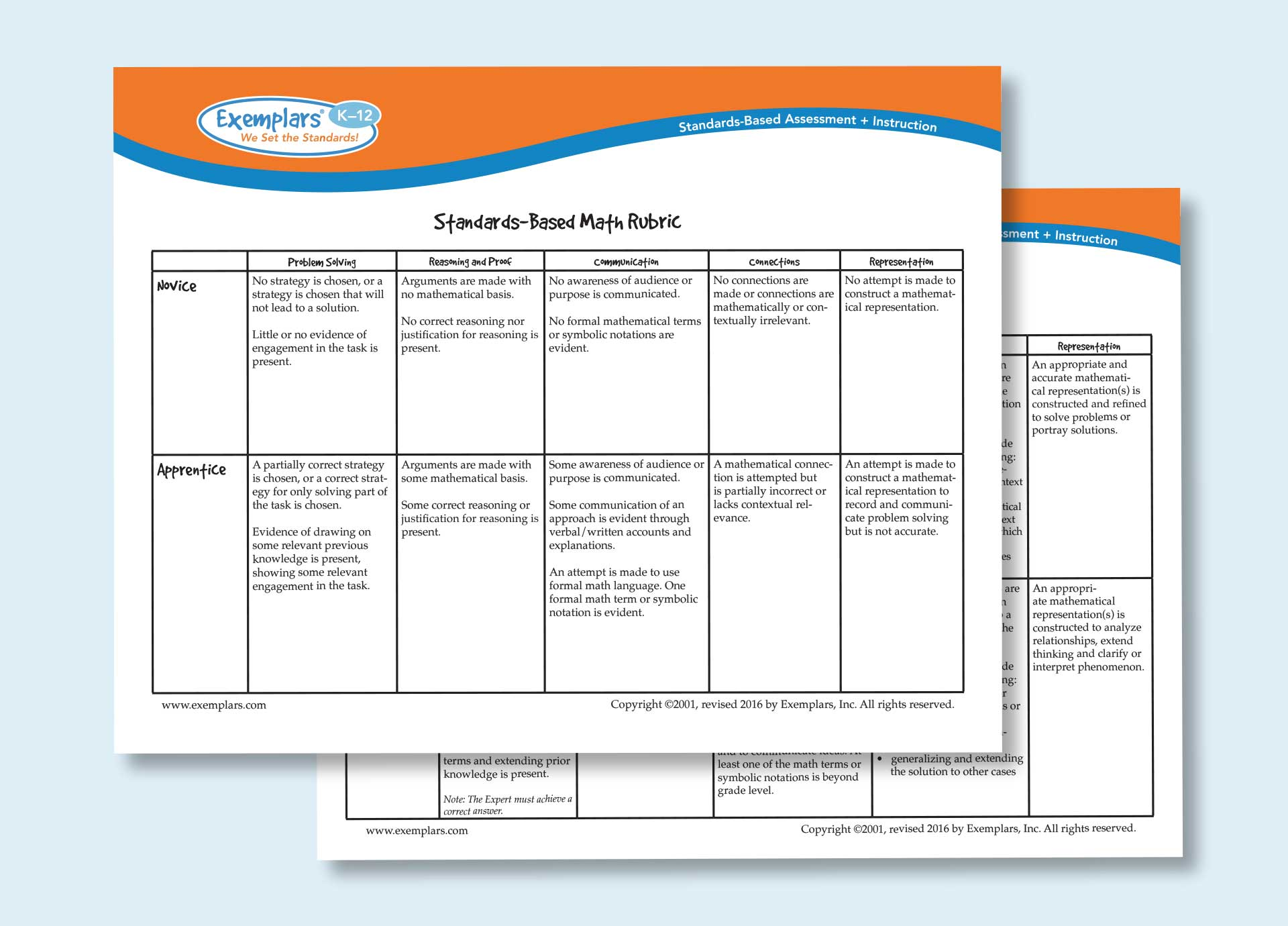Second Grade Student Solution - Sample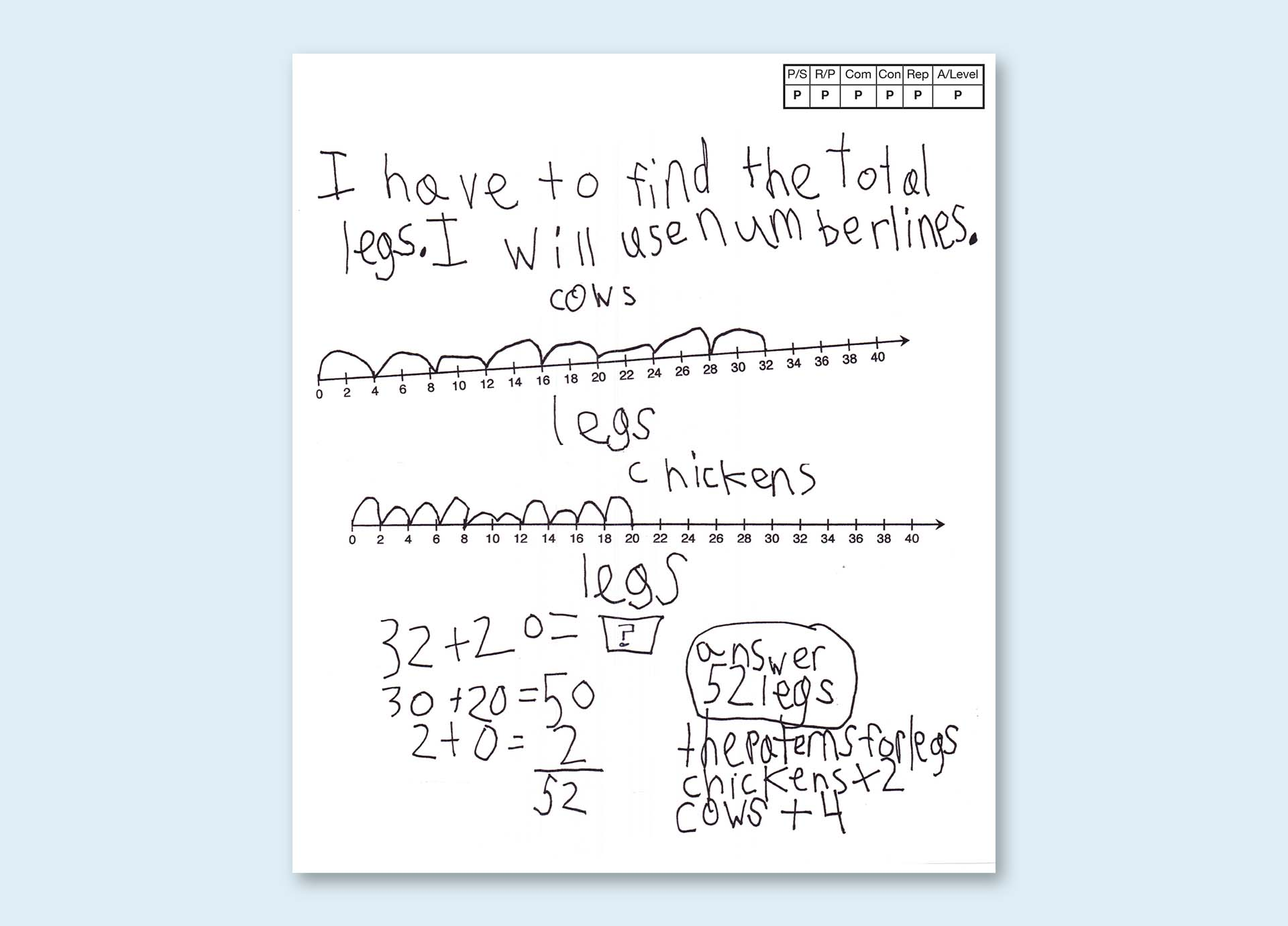#### Why is this important?

When we ask students to create solutions that demonstrate their mathematical understanding, we are also asking them to think more deeply and to develop a richer understanding of the mathematics they need to learn. Both the NCTM Process Standards and the Mathematical Practices underscore the value of rich solutions. A student’s solution also provides valuable formative assessment insights for teachers into the student’s grasp of math concepts and skills—insights that solving stand-alone calculations simply can’t deliver.

#### How can I help my students move from answers to solutions?

Every Exemplars task is built to elicit rich solutions. When students use the Exemplars Problem Solving Process and Student Rubric in concert with our tasks, they follow a process that results in a clear expression of their thoughts—and they practice connecting those thoughts to other mathematical ideas.

Here’s how the whole process works:

First, students need to read for understanding. Next, students should write an “I have to…” or “I need to..." sentence. This process of summarizing the overall task into a single sentence helps a student take ownership of the problem they will work to solve. This sentence explains what the problem is asking to be solved, in a student’s own words. This is asked for in the red section of the Problem-Solving Process.

Students needing support may have their “I need to...” sentence scribed by their teacher or any support person. Complete sentences are not required.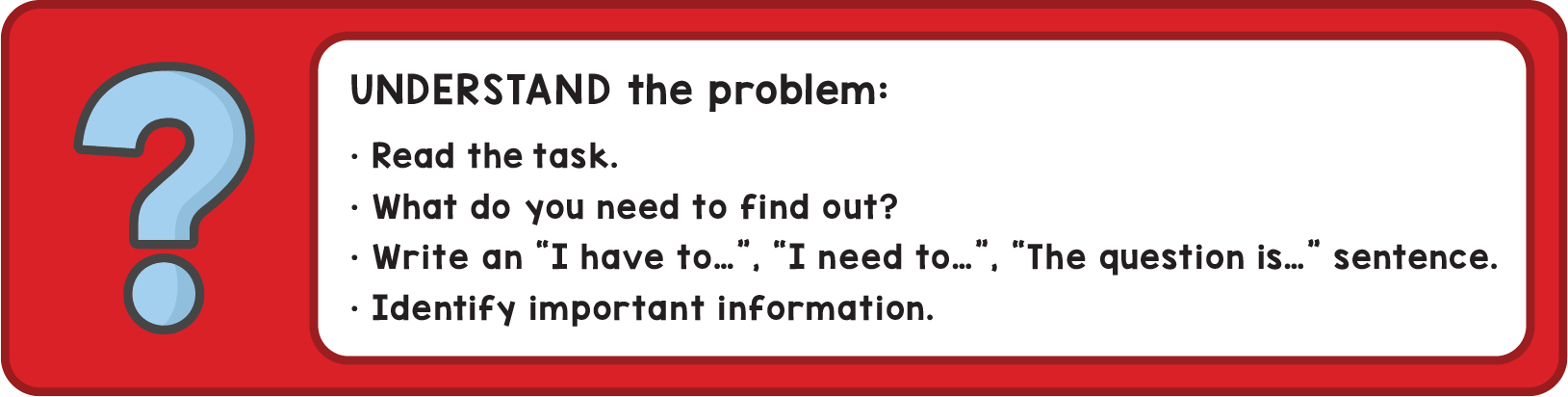Second, after spending time collaborating with their classmates or considering the task independently, students need to describe the strategy they will use to solve the problem. For this, students should write an “I will ...” , or “My strategy is …” sentence. For example: “I will use a diagram” or “I will use a bar graph” (table, graph, etc). This is asked for in the yellow section of the Problem-Solving Process sheet.

Students needing support may have their strategy scribed by their teacher or any support person. Complete sentences are not required.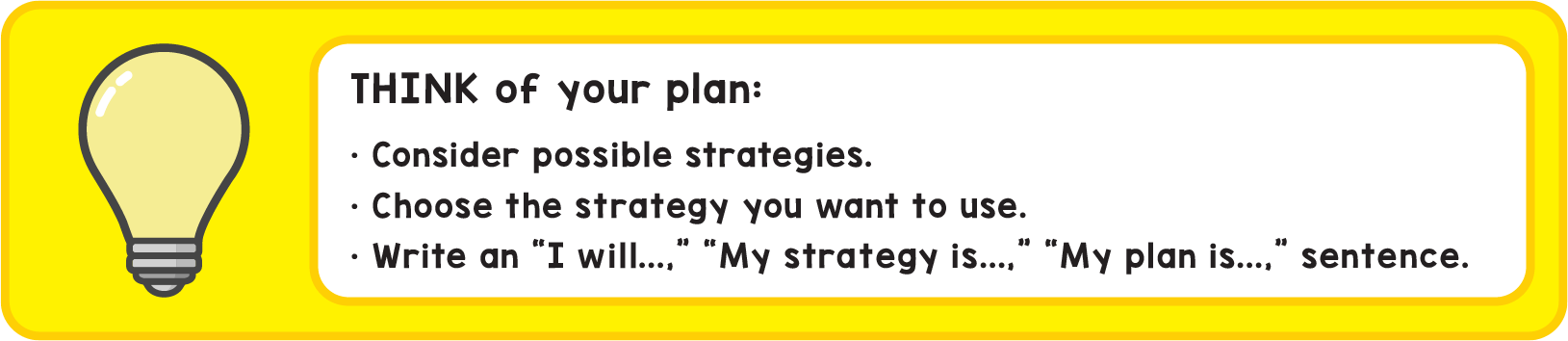Third, students should show the actual math strategy—a diagram, a number line, a table, a graph, and so on—they selected to solve the problem. Whatever their strategy, students need to show this within their work. This is also where students can include their calculations, equations, formulas, et cetera. A question for students to be thinking about is, “What calculations does a reader need to see to trust that the answer I have provided is correct?” This is the blue section of the Problem-Solving Process sheet.Fourth, students should use the Problem-Solving Process or Student Rubric to review their solutions and self-assess their work. They should reflect on these questions:

• Did I state an answer?
• Did I include at least two formal math terms and/or symbolic notation beyond basic numerals and calculation symbols?
• Are the representations appropriate, labeled, and accurate?

This is the green section of the colored Problem-Solving Process sheet. (Look for a deeper discussion of self-assessment on Day 5.)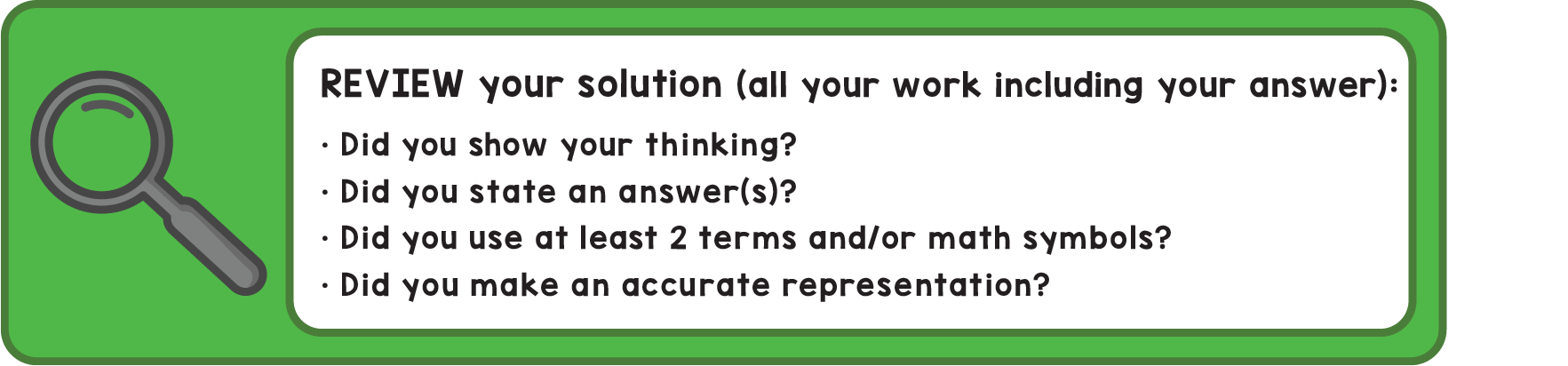Finally, students need to include a mathematical connection. Connecting today’s mathematical thinking with ideas students have learned in the past helps build a deep understanding of how all mathematical concepts fit together. Students can make a connection at any time while creating their solution. Some connections could include:

• How does this problem connect with the math concept they studied in past grades and in their current grade?
• Can the student use another representation to solve the problem?
• Can the student solve the problem another way?
• Does the student see any patterns?
• Can the student describe this pattern as a rule?

Students needing support may have their connection statements scribed by their teacher or any support person. Complete sentences are not required.

This is the purple section of the colored Problem-Solving Process sheet.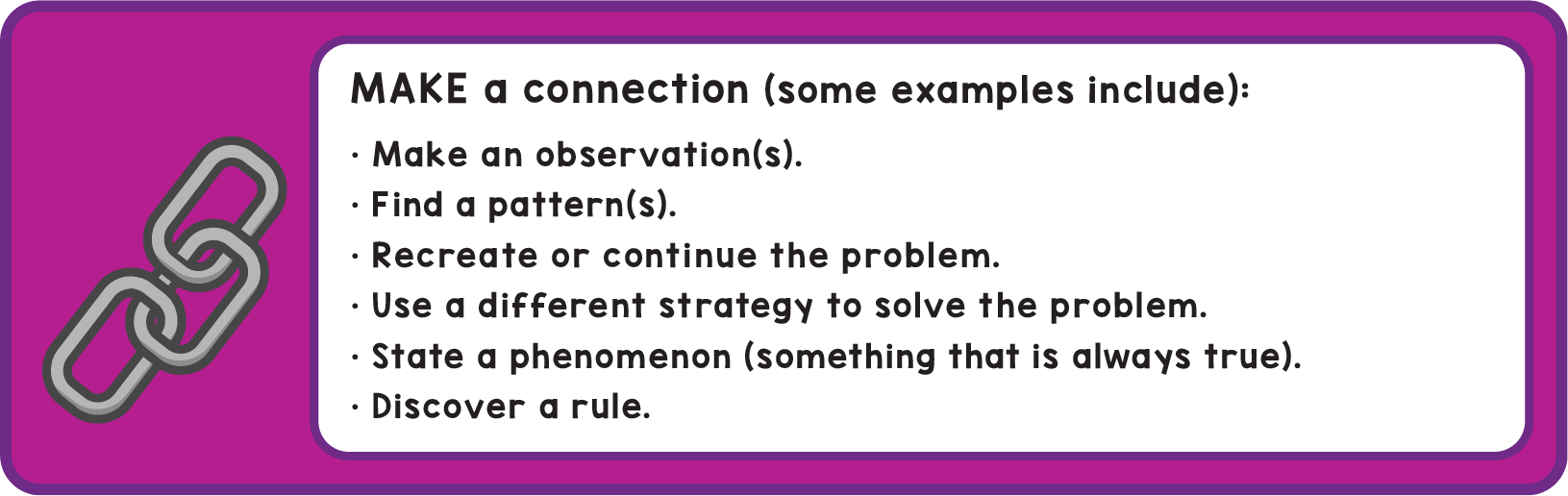Watch how we’d step a student through this powerful process and show them what a strong solution should look like:

When students follow this process from start to finish, they’re supported by a framework that’s designed to produce robust solutions. And when you help them practice it, they develop a durable habit that will let them solve complex problems in the classroom and beyond.

What comes after creating solutions? Reflecting on our work. So for Day 5 we’ll discuss the value of self- and peer-assessment, and explore some tools for supporting it in the classroom and online. To try these approaches in your classroom starting today, sign up for a free trial of the Exemplars Library. You can also request a quote or speak to us about your needs—or support your teachers’ skills through Exemplars professional development.

Topics: